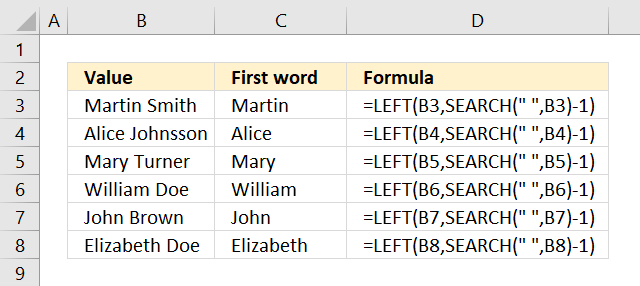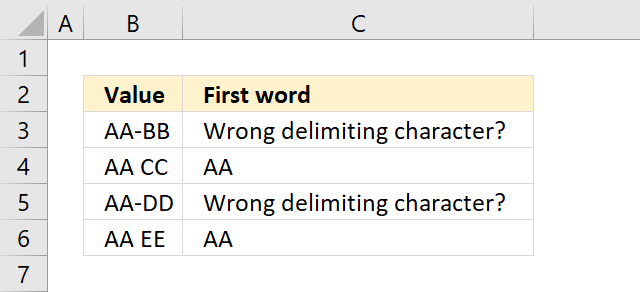Author: Oscar Cronquist Article last updated on January 22, 2022The formula in cell C3 grabs the first word in B3 using a blank as the delimiting character.

=LEFT(B3,SEARCH(" ",B3)-1)

The SEARCH function looks for a "space" character in cell B3 and returns 7, if you want to use a different delimiting character change the first argument in the SEARCH function.

We don't need the space character so we subtract the number returned from the SEARCH function with 1.

The LEFT function then extracts the first word in cell B3 using the calculated number.

### 1.1 Explaining formula

#### Step 1 - Find string in value

The SEARCH function returns a number representing the position of character at which a specific text string is found reading left to right.

SEARCH(find_text,within_text, [start_num])

SEARCH(" ", B3)-1

becomes

SEARCH(" ", "Martin Smith")-1

becomes

7-1 equals 6.

#### Step 2 - Extract string based on position

The LEFT function extracts a given number of characters always starting from the left.

LEFT(text, [num_chars])

LEFT(B3, SEARCH(" ", B3)-1)

becomes

LEFT(B3, 6)

becomes

LEFT("Martin Smith", 6)

and returns "Martin" in cell C3.

## 2. Extract the first word in cell - return warning if not found=IF(COUNT(SEARCH(" ",B3)), LEFT(B3,SEARCH(" ",B3)-1),"Wrong delimiting character?")

The SEARCH function returns #VALUE error if the delimiting character is not found. The COUNT function counts how many numbers are in a cell or cell range, it also ignores errors that come in handy in this case.

The COUNT function returns 0 (zero) in cell B3 and the IF function interprets that as a FALSE. The third argument in the IF function is returned "Wrong delimiting character?".

### 2.1 Explaining formula

#### Step 1 - Find position of character

The SEARCH function returns a number representing the position of character at which a specific text string is found reading left to right.

SEARCH(find_text,within_text, [start_num])

SEARCH(" ",B3)-1

becomes

SEARCH(" ","AA-BB")-1

becomes

#N/A! -1 and returns #N/A!.

#### Step 2 - Extract string from value

The LEFT function extracts a given number of characters always starting from the left.

LEFT(text, [num_chars])

LEFT(B3,SEARCH(" ",B3)-1)

becomes

LEFT(B3,#N/A!)

and returns #N/A!

#### Step 3 - Count numbers

The COUNT function counts all numerical values in an argument, it allows you to have up to 255 arguments.

COUNT(SEARCH(" ",B3))

becomes

COUNT(#N/A!)

and returns 0 (zero).

#### Step 4 - Show warning if the character is not found

The IF function returns one value if the logical test is TRUE and another value if the logical test is FALSE.

IF(logical_test, [value_if_true], [value_if_false])

IF(COUNT(SEARCH(" ",B3)), LEFT(B3,SEARCH(" ",B3)-1),"Wrong delimiting character?")

becomes

IF(0, LEFT(B3,SEARCH(" ",B3)-1),"Wrong delimiting character?")

and returns "Wrong delimiting character?" in cell C3.